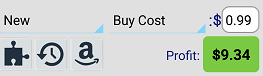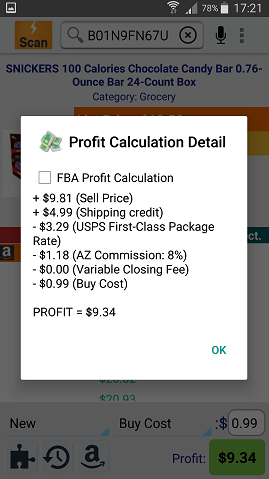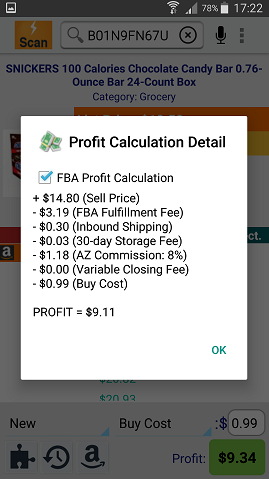# Understanding Profit Calculation For Profit Bandit On Android

Start by looking at the bottom of Profit Bandit's home screen.Starting from the left, you'll see:

• New - You can calculate your profit based on the item condition. Tap the "New" icon and a window will pop up with all the available condition options
• Buy Cost/Sell Price - You can edit the buy cost or the sell price by tapping on the "Buy Cost" box. You will get a screen where you can select between the two of them. In this case, the buy cost is set to \$0.99.
• Profit - This is where you'll see your estimated profit. If it's green, then Profit Bandit has calculated a Profit for the item you scanned. If it's red, then Profit Bandit predicts that you'll lose money if you sell the item with the buy cost and selling price that you have entered.

If you edit either the Buy \$ or Sell \$, then the Profit calculation will update in real time.

Tap the green/red Profit area to see exactly how Profit Bandit calculated your Profit/Loss.

MFN Profit Calculation                                            FBA Profit CalculationYou can switch between MFN and FBA profit calculations by checking/unchecking the box next to FBA Profit Calculation.

Be aware, however, that the default calculation shown on Profit Bandit's home screen will be the Seller Type you have selected in Profit Bandit's FBA Settings.

Not what you were looking for?